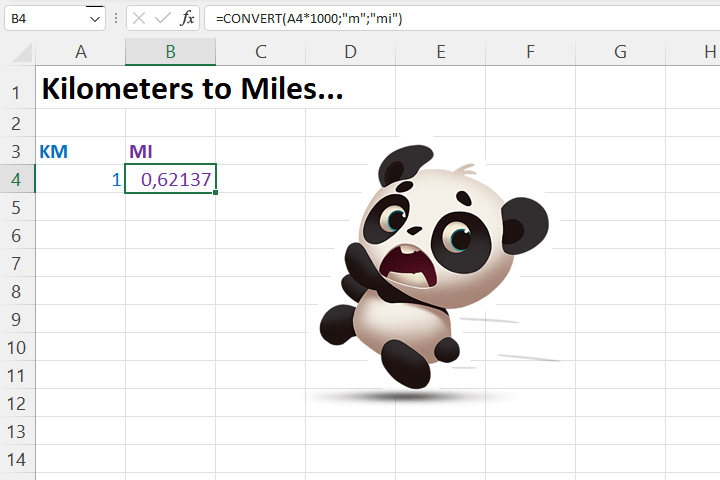# CONVERT function

How many grams are there in one cup? How many grams fit in a tablespoon? And in a coffee spoon? This is information that until now you had to search for on the Internet, and since recently there is an Excel function that enables these and some other conversions. It is very easy to use, and if you want to know how, read this “recipe” (it cannot be converted into several smaller ones, but it is interesting)!

To convert from one unit of measure to another, we use the CONVERT function. Its syntax:

CONVERT(<number>,<from unit>,<to unit>)

The first argument is the size to be converted. Here we can enter a number, expression or reference to a cell. The second argument is the unit of measure from which the conversion is made, and the last is the unit of measure to which we make the conversion.

For example, if we want to convert km to miles, we can achieve this using the formula:

=CONVERT(A4*1000,”m”,”mi”)

Vice-versa, converting miles to kilometers is achieved using the following formula:

=CONVERT(A4/1000,”mi”,”m”)